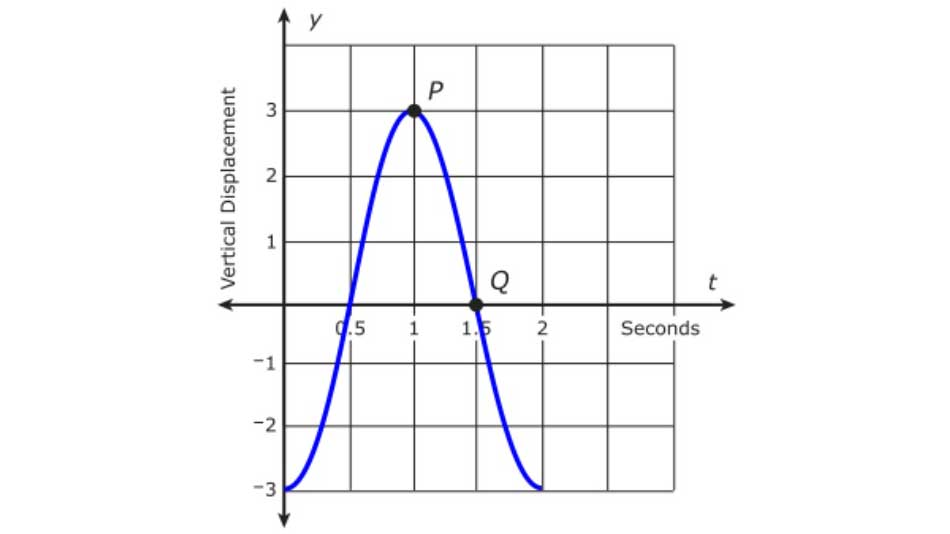Tuesday, October 20, 2020# Algebra 2 PARCC: Spring rate of change

-

The following fill-in-the-number question, explained here in hopes of helping algebra 2 students and their parents in Maryland prepare for the PARCC test near the end of this school year, appears on the released version of the PARCC Algebra 2 sample test released following the 2016 test (#3).Suppose that a weight is attached to a hanging spring. When the weight is pulled down and released, it will bob up and down with vertical displacement from its original resting point. The graph shows a weight pulled down 3 centimeters and allowed to go up and down for 2 seconds.Determine the average rate of change from Point P to Point Q.

The high school math standards in the Common Core, “Functions” section (HSF.IF.C.7.C), require students to be able to “calculate and interpret the average rate of change of a function (presented symbolically or as a table) over a specified interval,” and to “estimate the rate of change from a graph.”

The question is aligned neither with the strict language of the Common Core standard adopted by about 40 states nor with the spirit of those standards. See the analysis on this page for an explanation.

Sample solution strategy (there are others)

Use the graph and compute the “average” rate of change between two points.

The point P is at ordered pair (1, 3) and the point Q is at ordered pair (1.5, 0). The rate of change between those two points, regardless of what the function does in between the two points, is similar to finding the slope of a line.$m = \frac{\Delta y}{\Delta x} = \frac{y_{final}-y_{initial}}{x_{final} - x_{initial}}$$\frac{0-3}{1.5-1} = \frac{-3}{0.5} = -6$

## Analysis of this question and online accessibility

The question does not assess the algebra 2 skills of students, but as it is on the public release form, it would be considered fair game on the PARCC test, despite its misalignment to the Common Core standard referenced in PARCC’s alignment document.

First, the context is irrelevant and adds unnecessarily to the reading load of the question. The answer can be determined by using only the graph and completely ignoring the context of the spring. The graph is the second issue here: the y axis is not labeled in terms of what the 1, 2, and 3 mean. The text of the problem refers to centimeters, so that label can be deduced, but failure to print the units on the graph is an error in data representation, which violates the spirit of the Common Core.

In addition, a rate of change has units of something like distance per unit time. No label is provided in the answer, and in this case, no units are provided in the text, either. As this is the case—the student is not required to enter an answer in cm/s—a student could technically enter .06 and claim, after the fact that his answer was entered in m/s and is the correct rate of change. This is an oversight on the part of the test’s editors or question writers, and means that there is more than one correct answer, given the absence of units.

As a result of the above, the question is invalid. That doesn’t mean you shouldn’t prepare to answer questions that ask you to find the rate of change. The Common Core technically says you only need to compute an exact rate of change for a symbolic or tabular representation of a function while only making an “estimate” of the rate of change for a graph.

But as we have pointed out, the PARCC people apparently have a reading comprehension problem when reading the English words in the Common Core standards. The following are required for high school algebra 2:

• Compute an exact rate of change from a tabular or symbolic representation of a function
• Estimate a rate of change from a graphical representation of a function

The question can be delivered online or on paper. However, because students have to type in an answer online, a multiple-choice question would ordinarily be substituted for this question on a paper-based test. A multiple-choice question, because one-fourth of the students who don’t know anything about the subject can be expected to guess the correct answer, would have slightly different performance statistics from the online version of the item, which greatly reduces the ability of students to guess the correct answer.

Be prepared for this type of question, since it’s on the released form, despite a slight misalignment with the technical language in the Common Core.

No special accommodation challenges can be identified with this question, so the question is considered fair.Paul Katulahttp://news.schoolsdo.org
Paul Katula is the executive editor of the Voxitatis Research Foundation, which publishes this blog. For more information, see the About page.

### Atlanta closes to in-person through year’s end

0
Public schools in Atlanta will continue e-learning through at least the end of December, due to a rise in Covid-19 cases in the community.